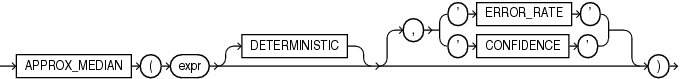## APPROX_MEDIAN

SyntaxDescription of the illustration approx_median.eps

Purpose

`APPROX_MEDIAN` is an approximate inverse distribution function that assumes a continuous distribution model. It takes a numeric or datetime value and returns an approximate middle value or an approximate interpolated value that would be the middle value once the values are sorted. Nulls are ignored in the calculation.

This function provides an alternative to the `MEDIAN` function, which returns the exact middle value or interpolated value. `APPROX_MEDIAN` processes large amounts of data significantly faster than `MEDIAN`, with negligible deviation from the exact result.

For `expr`, specify the expression for which the approximate median value is being calculated. The acceptable data types for `expr`, and the return value data type for this function, depend on the algorithm that you specify with the `DETERMINISTIC` clause.

DETERMINISTIC

This clause lets you specify the type of algorithm this function uses to calculate the approximate median value.

• If you specify `DETERMINISTIC`, then this function calculates a deterministic approximate median value. In this case, `expr` must evaluate to a numeric value, or to a value that can be implicitly converted to a numeric value. The function returns the same data type as the numeric data type of its argument.

• If you omit `DETERMINSTIC`, then this function calculates a nondeterministic approximate median value. In this case, `expr` must evaluate to a numeric or datetime value, or to a value that can be implicitly converted to a numeric or datetime value. The function returns the same data type as the numeric or datetime data type of its argument.

ERROR_RATE | CONFIDENCE

These clauses let you determine the accuracy of the value calculated by this function. If you specify one of these clauses, then instead of returning the approximate median value for `expr`, the function returns a decimal value from 0 to 1, inclusive, which represents one of the following values:

• If you specify `ERROR_RATE`, then the return value represents the error rate for the approximate median value calculation for `expr`.

• If you specify `CONFIDENCE`, then the return value represents the confidence level for the error rate that is returned when you specify `ERROR_RATE`.

• MEDIAN

• APPROX_PERCENTILE which returns, for a given percentile, the approximate value that corresponds to that percentile by way of interpolation. `APPROX_MEDIAN` is the specific case of `APPROX_PERCENTILE` where the percentile value is 0.5.

Examples

The following query returns the deterministic approximate median salary for each department in the `hr`.`employees` table:

```SELECT department_id "Department",
APPROX_MEDIAN(salary DETERMINISTIC) "Median Salary"
FROM employees
GROUP BY department_id
ORDER BY department_id;

Department Median Salary
---------- -------------
10          4400
20          6000
30          2765
40          6500
50          3100
60          4800
70         10000
80          9003
90         17000
100          7739
110          8300
7000```

The following query returns the error rates for the approximate median salaries that were returned by the previous query:

```SELECT department_id "Department",
APPROX_MEDIAN(salary DETERMINISTIC, 'ERROR_RATE') "Error Rate"
FROM employees
GROUP BY department_id
ORDER BY department_id;

Department Error Rate
---------- ----------
10 .002718282
20 .021746255
30 .021746255
40 .002718282
50 .019027973
60 .019027973
70 .002718282
80 .021746255
90 .021746255
100 .019027973
110 .019027973
.002718282```

The following query returns the confidence levels for the error rates that were returned by the previous query:

```SELECT department_id "Department",
APPROX_MEDIAN(salary DETERMINISTIC, 'CONFIDENCE') "Confidence Level"
FROM employees
GROUP BY department_id
ORDER BY department_id;

Department Confidence Level
---------- ----------------
10       .997281718
20       .999660215
30       .999660215
40       .997281718
50       .999611674
60       .999611674
70       .997281718
80       .999660215
90       .999660215
100       .999611674
110       .999611674
.997281718```

The following query returns the nondeterministic approximate median hire date for each department in the `hr`.`employees` table:

```SELECT department_id "Department",
APPROX_MEDIAN(hire_date) "Median Hire Date"
FROM employees
GROUP BY department_id
ORDER BY department_id;

Department Median Hire Date
---------- ----------------
10        17-SEP-03
20        17-FEB-04
30        24-JUL-05
40        07-JUN-02
50        15-MAR-06
60        05-FEB-06
70        07-JUN-02
80        23-MAR-06
90        17-JUN-03
100        28-SEP-05
110        07-JUN-02
24-MAY-07```